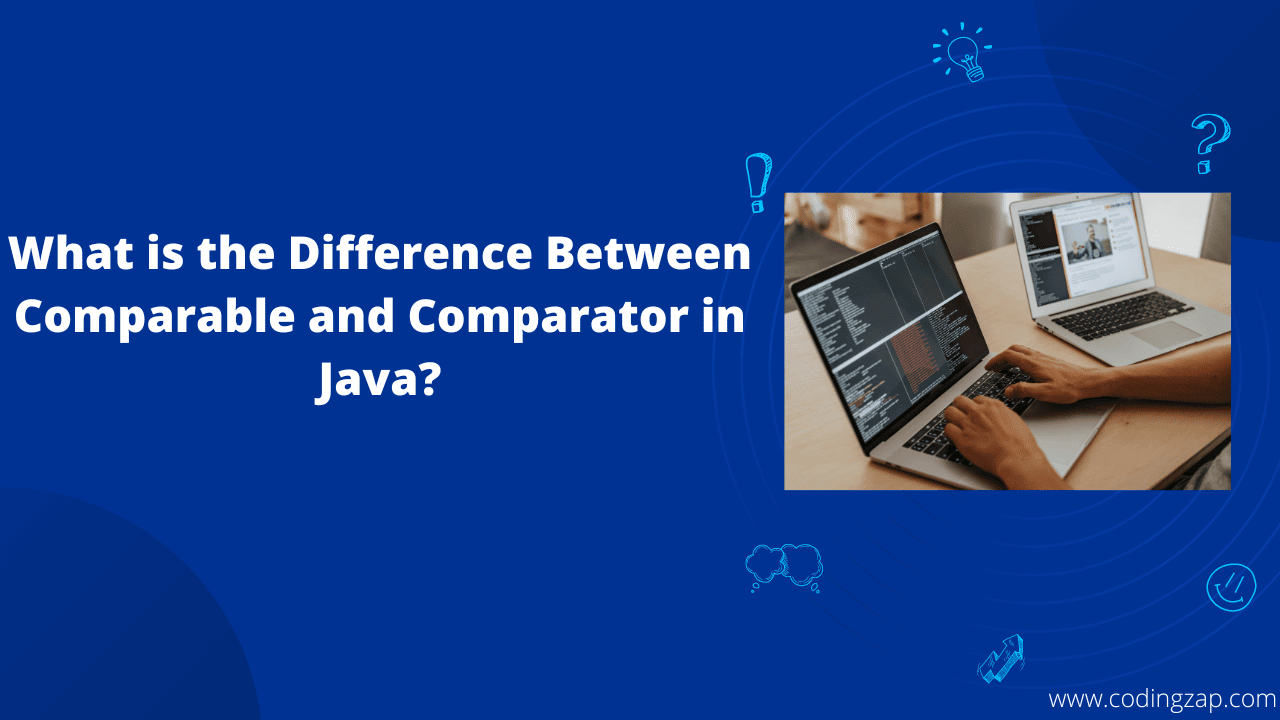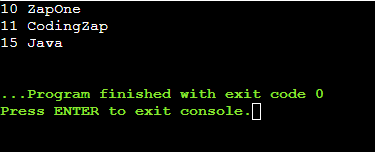# Difference Between Comparable and Comparator in Java

Do you know what are the differences between the Comparable vs Comparator in Java? Well, do you have any idea about how Comparators work, how Comparable works? Have you ever thought about what is called a Comparable and comparator in Java? So, if you’re interested in getting Help with Java homework and curious to know the difference between Comparable and Comparator in Java then this article is for you.

Let us know some interesting facts while learning about the Comparable vs Comparator in Java. In this article, we will try to clarify the concept related to Comparable vs Comparator Java. Also, along with that, we will try to implement Comparable & Comparator in Java. This will help to know how comparators work.

But before we start the topic, let us understand the background of it with a simple example. Let us assume one scenario.

Suppose, if have two friends. They both are good to sort things in a particular order. But one of your friends is weaker than the other one to sort anything. He can only sort a particular thing at a time. Whereas your other friend can sort many items at a certain time.

Now, you need to sort three items in a very small time. Whom will you ask to help you in such a case?

You will ask the second friend who can sort many items at the same time. This is the basic background of Comparable vs Comparator Java. Keep this theory in mind. It will help to understand the topic in a good manner.

But before we start making the differences between Comparable vs Comparator in Java, let us first know about them briefly.

## What Is Comparable?

There are two interfaces in Java for sorting purposes. In between them, Comparable is an important one. Sorting any one type of data in Java is a very easy task. But when we need to sort the data into different data types, it becomes a difficult one. When we need to compare a set of things by any of the data, this interface can help.

Comparable is like your friend who can sort any one item at a given time. Comparable can be used to sort the lists of elements by using any one item. It is a very easy sorting interface. Comparable uses the compareTo() method. It is the same method that we used to compare two strings in Java. If you’re not aware about how to compare strings in Java, then you can read it here. Also, in this case, while sorting it modifies the original class elements. That may create problems at some point in time.

Many developers used it for sorting such cases. But it has one drawback, it can’t able to sort the list by the many data types at the same time. For that purpose, we need to take the help of a Comparator. Also, if you wan to know more about algorithms then you can check out our article on “Quick Sort Algorithm” to have more knowledge about the same.

## What Is Comparator?

Comparator is another sorting interface in Java. It also uses for the same purpose as the Comparable. It is also used for sorting the list of elements. Comparator sorts the elements by the different types of data types at the same time. It consumes a small amount of time to complete the process.

A Comparator is like your other friend. The friend who can sort many items at a certain time. As Comparator can sort the items in a certain time, many developers implement Comparator in their code. It is quite difficult compared to the Comparable method. it uses the compare() method. This method is difficult to implement. Also, it doesn’t change the original class elements there.

We have gained some knowledge about Comparable & Comparator. Now, it is time to get some more knowledge by making a table of differences between Comparable vs Comparator.

Let us develop the table & acquire some more knowledge from it.

## What is the Differences Between The Comparable vs Comparator?

 Comparable In Java Comparator In Java It can sort the list with the help of only one field at the same time. Comparator can sort the list with the help of many fields at the same time. It changes the original class elements. The actual class is being modified here. It doesn’t change the original class. The actual class remains the same as earlier. Java.lang package should be used to implement Comparable. Java.utill package should be used to implement Comparator. It uses the compareTo() method to compare two fields & sort them. It uses the compare() method to compare two fields & sort them. General Syntax: Collections.sort(listname); General Syntax: Collections.sort(listname, comparator)

## How to Do Implementation Of Comparable In Java?

After we know the differences between the Comparable vs Comparator, it is time to know how comparable works. For knowing that, we have to implement a Comparable code. We have to develop two important parts there.

• Declaration Of Comparable Class: Here, we need to declare a class that will act as the Comparable. Here, we need to provide the fields. We can add different fields of different data types. Here we have added two fields. One is the Id which takes an integer value. Another is Name which takes a string value. The values which will be provided by the user will store here.

```// Declaration Of Comparable Class

class experiment implements Comparable<experiment>{

// Declaring The Fields

int id;

String name;

experiment(int id,String name){

this.id=id;

this.name=name;}

```
• Comparing The Field: Here, we need to compare the lists by a certain field. Here, we have taken the Ids for comparing purposes. If the certain id is greater than the first one, it will return 1. Otherwise, if it is smaller then it will return the -1. In this way, we have to compare the items. Also, based on the operation sorting will be completed.

```// Class To Compare Between The Inputs Based On The Ids

public int compareTo(experiment ex){

if(id==ex.id)

return 0;

else if(id>ex.id)

return 1;

else

return -1;}

```

Above are the code snippets. If needed, you can go through the full source code provided here. This will help to clear the differences between Comparable vs Comparator. The probable output of the above code will be the following.

Output:## How to Do Implementation Of Comparator In Java?

Now, it is time to know how Comparators work. In this case, also, we need to first develop the Comparator class. The implementation of the Comparator class will be the same as for the Comparable class.  Here, the Comparator can sort the list by many fields at the same time. Here, we have to sort the list by the Id & the Name field.Also, If you are interested in learning another topic of implementation in Java then read this article about priority queue in Java and what will be the process to implement priority queue?

• Comparing The Field By Id: Here the logic is the same as for the Comparable. But here, we have to consider the values of both entities. Then we have to compare it. If the first id is greater than the second one, it will return 1. Otherwise, if it is smaller than a second will return the -1. In this way, we have to compare the items. Also, based on the operation sorting will be completed.

```// Program For Id Comparator

class IdComparator implements Comparator<experiment>{

public int compare(experiment s1,experiment s2){

if(s1.id==s2.id)

return 0;

else if(s1.id>s2.id)

return 1;

else

return -1; }

```
• Comparing The Field By Name: In this case, we have to compare two names. Then we have to sort it out. As the names are the strings, we can use the compareTo() method. We have already seen the use of this method in the article comparing two strings in Java. It will compare & returns some arbitrary value. Based on it, Java sorts the names in ascending order.

```// Class For Name Comparator

class NameComparator implements Comparator<experiment>{

public int compare(experiment s1,experiment s2){

return s1.name.compareTo(s2.name);  }  }

```

Above are the code snippets. If needed, you can go through the full source code provided here. This will help to clear the differences between Comparable vs Comparator. The probable output of the above code will be the following.

Output:## Conclusion:

As we saw differences between Comparable vs Comparator are very important.

We have to clear the basics of the Java programming language to understand the concept in a good manner.

As per the choice of the individual, they can use any method mentioned above. It is a very useful implementation.

So, hope you have liked this piece of article. Share your thoughts in the comments section and let us know if we can improve more.

Also, if you are looking for Final Year Java Project Ideas then you can contact us as well.